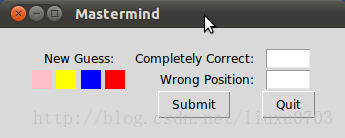A-A+

# [Tkinter Gui 教程13] Mastermind 游戏

2019年12月20日 11:31 阅读 290 views 次

## Mastermind 游戏## 实现代码

``````from tkinter import *
from tkinter.messagebox import *
import random

from combinatorics import all_colours

def inconsistent(p, guesses):
""" the function checks, if a permutation p, i.e. a list of
colours like p = ['pink', 'yellow', 'green', 'red'] is consistent
with the previous colours. Each previous colour permuation guess
compared (check()) with p has to return the same amount of blacks
(rightly positioned colours) and whites (right colour at wrong
position) as the corresponding evaluation (guess in the
list guesses) """
for guess in guesses:
res = check(guess, p)
(rightly_positioned, permutated) = guess
if res != [rightly_positioned, permutated]:
return True # inconsistent
return False # i.e. consistent

""" checking of an evaulation given by the human player makes
sense. 3 blacks and 1 white make no sense for example. """
(rightly_positioned, permutated) = a
if (rightly_positioned + permutated > number_of_positions) \
or (rightly_positioned + permutated < len(colours) - number_of_positions):
return False
if rightly_positioned == 3 and permutated == 1:
return False
return True

def get_evaluation():
""" get evaluation from entry fields """
rightly_positioned = int(entryWidget_both.get())
permutated = int(entryWidget_only_colours.get())
return (rightly_positioned, permutated)

def new_evaluation(current_colour_choices):
""" This funtion gets an evaluation of the current guess, checks
the consistency of this evaluation, adds the guess together with
the evaluation to the list of guesses, shows the previous guesses
and creates a ne guess """
rightly_positioned, permutated = get_evaluation()
if rightly_positioned == number_of_positions:
return(current_colour_choices, (rightly_positioned, permutated))

print("Input Error: Sorry, the input makes no sense")
return(current_colour_choices, (-1, permutated))
guesses.append((current_colour_choices, (rightly_positioned, permutated)))
view_guesses()

current_colour_choices = create_new_guess()
show_current_guess(current_colour_choices)
if not current_colour_choices:
return(current_colour_choices, (-1, permutated))
return(current_colour_choices, (rightly_positioned, permutated))

def check(p1, p2):
""" check() calcualtes the number of bulls (blacks) and cows (whites)
of two permutations """
blacks = 0
whites = 0
for i in range(len(p1)):
if p1[i] == p2[i]:
blacks += 1
else:
if p1[i] in p2:
whites += 1
return [blacks, whites]

def create_new_guess():
""" a new guess is created, which is consistent to the
previous guesses """
next_choice = next(permutation_iterator)
while inconsistent(next_choice, guesses):
try:
next_choice = next(permutation_iterator)
except StopIteration:
return ()
return next_choice

def new_evaluation_tk():
global current_colour_choices
res = new_evaluation(current_colour_choices)
current_colour_choices = res

def show_current_guess(new_guess):
row = 1
Label(root, text="   New Guess:   ").grid(row=row,
column=0,
columnspan=4)
row +=1
col_count = 0
for c in new_guess:
print(c)
l = Label(root, text="    ", bg=c)
col_count += 1

def view_guesses():
row = 3
Label(root, text="Old Guesses").grid(row=row,
column=0,
columnspan=4)
Label(root, text="c&p").grid(row=row,
column=number_of_positions + 1)
Label(root, text="p").grid(row=row,
column=number_of_positions + 2)
# dummy label for distance:
Label(root, text="         ").grid(row=row,
column=number_of_positions + 3)

row += 1
# vertical dummy label for distance:
Label(root, text="             ").grid(row=row,
column=0,
columnspan=5)

for guess in guesses:
guessed_colours = guess
col_count = 0
row += 1
for c in guessed_colours:
print(guessed_colours[col_count])
l = Label(root, text="    ", bg=guessed_colours[col_count])
col_count += 1
# evaluation:
for i in (0,1):
l = Label(root, text=str(guess[i]))
l.grid(row=row,column=col_count + i + 1, padx=2)

if __name__ == "__main__":
colours = ["red","green","blue","yellow","orange","pink"]
guesses = []
number_of_positions = 4

permutation_iterator = all_colours(colours, number_of_positions)
current_colour_choices = next(permutation_iterator)

new_guess = (current_colour_choices, (0,0) )

row_offset = 1
root = Tk()
root.title("Mastermind")

entryLabel = Label(root)
entryLabel["text"] = "Completely Correct:"
entryLabel.grid(row=row_offset,
sticky=E,
column=number_of_positions + 4)
entryWidget_both = Entry(root)
entryWidget_both["width"] = 5
entryWidget_both.grid(row=row_offset, column=number_of_positions + 5)

entryLabel = Label(root)
entryLabel["text"] = "Wrong Position:"
entryLabel.grid(row=row_offset+1,
sticky=E,
column= number_of_positions + 4)
entryWidget_only_colours = Entry(root)
entryWidget_only_colours["width"] = 5
entryWidget_only_colours.grid(row=row_offset+1, column=number_of_positions + 5)

submit_button = Button(root, text="Submit", command=new_evaluation_tk)
submit_button.grid(row=4,column=number_of_positions + 4)

quit_button = Button(root, text="Quit", command=root.quit)
quit_button.grid(row=4,column=number_of_positions + 5)
show_current_guess(current_colour_choices)

root.mainloop()``````

## 相关tkinter 教程系列

### 布施恩德可便相知重

#### 微信扫一扫打赏#### 支付宝扫一扫打赏×